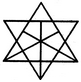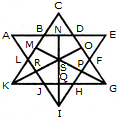# Non Verbal Reasoning - Analytical Reasoning - Discussion

Discussion Forum : Analytical Reasoning - Section 1 (Q.No. 11)
11.

Find the number of triangles in the given figure.21
23
25
27
Explanation:

The figure may be labelled as shown.The simplest triangles are ABL, BCD, DEF, FGP, PGH, QHI, JQI, KRJ and LRK i.e. 9 in number.

The triangles composed of two components each are OSG, SGQ, SPI, SRI, KSQ, KMS, FGH, JHI and JKL i.e. 9 in number.

There is only one triangle i.e. KSG which is composed of four components.

The triangles composed of five components each are NEI, ANI, MCG and KCO i.e. 4 in number.

The triangles composed of six components each are GMK and KOG i.e. 2 in number.

There is only one triangle i.e. AEI composed of ten components.

There is only one triangle i.e. KCG composed of eleven components.

Therefore, Total number of triangles in the given figure = 9 + 9+1 + 4 + 2+1 + 1 = 27.

Discussion:
6 comments Page 1 of 1.

PRANAY said:   2 years ago
Please anyone explain this in detail.

Rajashree said:   4 years ago
How to solve this?

Vivek said:   5 years ago
The total outer nodes+ inner node * 2 + 2.
13 + 2 * 6 + 2 = 27.

Suraj tharu said:   5 years ago